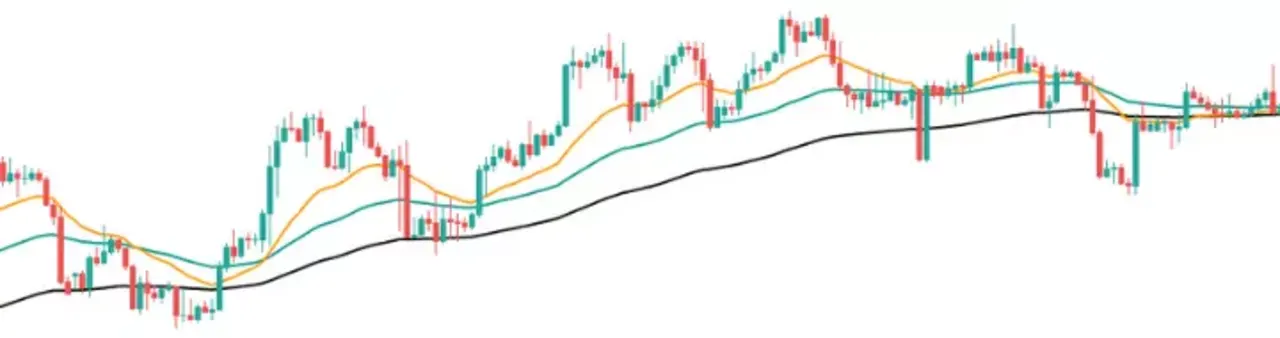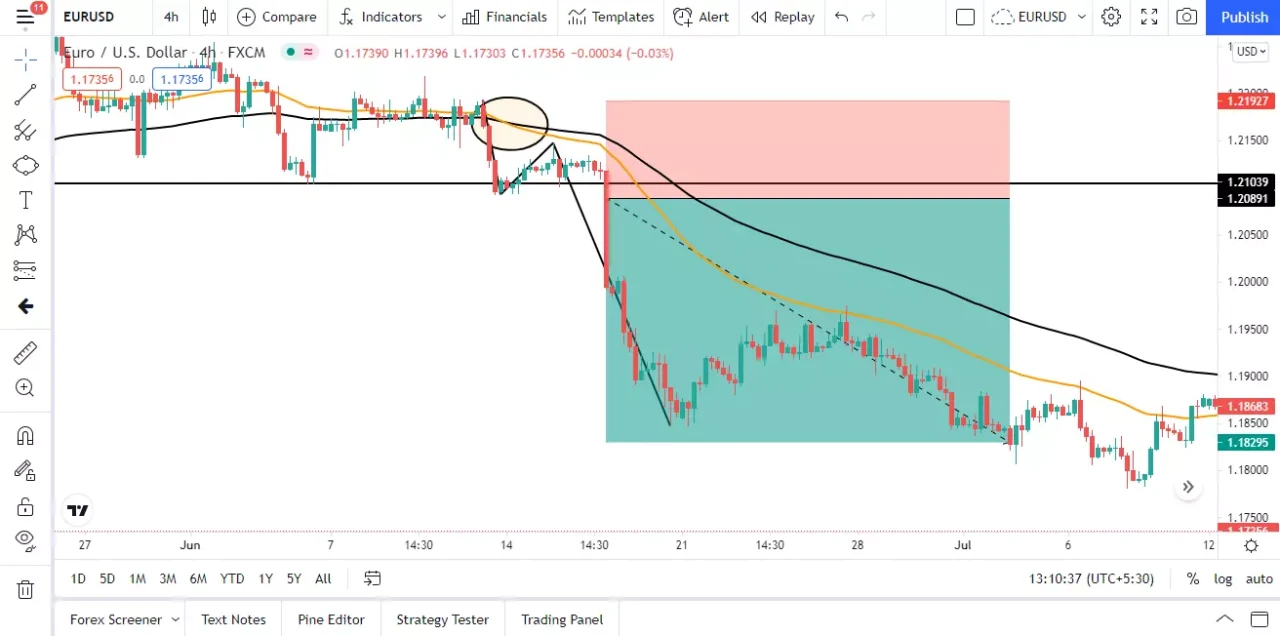# Moving Average: Detailed Guide

Moving averages are one of the most commonly used technical indicators by traders around the world and I am sure that you also might be knowing a thing or two about moving averages.

But, do you know about the most common types of moving averages, why should you use them in trading, and most importantly how should you use them in trading? Read this blog post to find all answers.

In this blog post, I am going to tell you everything you need to know about moving averages and you can start using it right away in trading.

Stick till the end as I will be discussing some moving average strategies that can help you capitalize on the price swings, so don’t skip any part.

### Contents

What are moving averages?

Types of moving averages

Why should you use moving averages?

Moving averages - The deal breakers

Which moving average do you use?

## What are moving averages?The concept of average is known to everyone. Average is basically the arithmetic mean of a given set of digits. In order to find an average, we first add all the digits in the given, the result is then divided by the number of digits that was present in the set.

This then gives us the average of the set. The same method is applied in moving averages. Moving averages are an extension of the concept of averages.

Let us consider the closing price of the USDINR currency pair for the last 5 days - 73.6750, 73.5100, 73.3000, 73.5000, and 73.7000.

In order to find the average closing price of the USDINR currency pair you would first add these up, which sums up to 367.6850, this would then be divided by the number of days i.e. 5, the result is 73.5370.

This is the average closing price of the USDINR currency pair for the last 5 days.

But what if you wanted to take the average of the last 5 close prices at the end of each trading session? You cannot manually keep calculating this every day for every currency pair right.

This is where moving averages are used. Moving averages give traders the continuous average price of the currency pair over the specified time period.

By continuous, it means that after every trading session the moving average discards the first digit in the set, or the oldest price of the currency pair, and includes the latest price of the currency pair into the set and then displays the average.

Let’s consider the above-mentioned example again, closing prices of 5 days of the USDINR currency pair are given - 73.6750, 73.5100, 73.3000, 73.5000, and 73.7000. The average is 73.5370.

But after the current trading session, the closing price is 73.8500. We want to find the average closing price of the last 5 trading sessions, hence we will discard the oldest closing price in the set i.e. 73.6750, and we will include the latest closing price in the set i.e. 73.8500.

The new set now is - 73.5100, 73.3000, 73.5000, 73.7000 and 73.8500. The average price of this set is 73.5720. This is what continuous average means.

Now imagine when this applied to closing price data of say 300 trading sessions. We will get the average closing prices every day for the last 5 days.

If you mark these average prices on a price chart it would seem like a series of random points on a chart, but when these points are joined and the resulting line is smoothened out a little you will get what is known as a moving average, with a period of 5 days. This is also called a 5-day moving average.

The number of days could vary, it could be as minimum as 2, as to find average we need a minimum of two entries, and could extend up to 500 days, 1000 days, or even more, as the trader requires.

In the given illustrations I have included only closing prices of the currency pair, but his concept of moving average can be applied to even the highest or lowest price of the day or to the open price of the day.

Moving averages can be applied to time frames greater than daily i.e. weekly and monthly, in order to find the weekly or monthly average price of the currency.

It could also be applied to timeframes lower than the daily i.e. to 4H, 1H, 30min, 15min, 5 min, 3min and can even be applied to charts that represent price movements that happen every second.

There is no limitation on the scope of calculation of moving averages. This is the reason for it being a popular and widely used technical tool.

## Types of moving averagesThere are many types of moving averages that have been developed in order to assist traders to take trading decisions.

These moving averages would differ in the method of calculating the given set of data.

The most commonly used moving averages are the Simple Moving Average and the Exponential Moving Average.

### 1. Simple Moving Average

This is the most basic type of moving average. It is basic because of the basic nature of its calculation.

Simple Moving Averages or SMA would give the average of the price over the specified time period. All prices are given the same weightage as the other price no matter how old or latest it is.

The calculation of the Simple Moving Average is the same as the calculation illustrated in the above examples.

The sum of all prices in that time period is divided by the time period. As and when new prices are formed, the oldest price from the set is discarded and the latest price is added to the set.

Here the price which was previously the second oldest now becomes the oldest.

This is repeated over time with new prices added and oldest prices excluded, and we have a continuous smooth line plotted on the chart which is known as a Simple Moving Average.

### 2. Exponential Moving Average

The Exponential Moving Average or EMA is a bit different from the Simple Moving Average with respect to the calculation.

The Exponential Moving Averages give more weightage to the latest price. This is done so that it is more responsive to the latest information.

For the calculation of Exponential Moving Average, the Simple Moving Average of the particular time period is calculated, and then a multiplier is calculated, this multiplier is not a constant but varies for each time period.

In the end, these two are used in the final calculation for the Exponential Moving Average.

The exact calculations are a bit complex to understand and since this blog post is related to trading and not related to statistics I will not get into the calculations part of EMAs.

There are more types of moving averages other than the Simple Moving Average and the Exponential Moving Average but these two are the most commonly used moving averages.

The other types of moving averages have different calculations and give varying weightage to the latest prices in the given set.

## Why should you use moving averages?Traders use moving averages in order to find the trend of the price of the currency. By merely seeing the price on a chart it might not give the trader many ideas about the trend of the price of the currency.

Since the moving average is a smooth and sloping line, it allows the trader to determine the trend and plan trades accordingly.

The various types of moving averages available to us are also an advantage. You can use all types and then decide which one works best for your trading plan.

If you just want to get a plain idea about the trend of price then you can use the Simple Moving Average.

If you want to know the trend but also want to know how the latest price has fluctuated then you can use the Exponential Moving Average.

## Moving averages - The deal breakersMoving averages are widely used technical indicators but it also comes with some criticisms. Moving averages face most criticism due to it being a lagging indicator.

Lagging indicators are those indicators that are based on price movement that has already happened. It is argued that moving averages only show what has happened in the past.

Since moving averages are based on the price of a currency alone, they do not depict the volatility of the price and they also do not predict market behavior.

Moving averages being a trend indicator, experience lots of whipsaws when the price is moving in a range, hence it becomes difficult to trade them during sideways movement.

One argument about the disadvantage of moving average is that since it is just an indicator with some calculations applied on price, it cannot predict the future price movements and cannot take into consideration the various external factors that affect the price movement of currencies.

## How to trade moving averages?

There are some popular methods that traders use in order to trade moving averages.

It should be noted that these methods are not exhaustive and that you can always think outside the scope of these methods and experiment on ways to use moving averages.

### 1. Moving average crossoversIn this method traders use two moving averages, one moving average will be of a shorter time period while the other will be of a longer time period.

The idea is to enter into trades when the shorter time period moving average crosses the longer time period moving average.

When the shorter time period moving average crosses the longer time period moving average on the upside, then traders enter into long positions.

This indicates that the current trend is becoming stronger and once the crossover takes place it means that the price will see a bullish move.

When the shorter time period moving average crosses the longer time period moving average on the downside, then traders enter into short positions.

This indicates that the current trend is becoming weaker and once the crossover takes place it means that the price will see a bearish move.

### 2. Moving average support and resistanceIt is often noticed that at times prices of currencies have difficulty in breaking above or below a moving average and bounces away from that particular moving average.

In such scenarios, traders first establish the prevailing trend of the price of the currency, they then look for any price pullbacks towards the moving averages.

You can then enter into trades in the direction of the prevailing trend of the price as the pullbacks are merely short-term minor price movements.

Many traders use moving averages like 20SMA, 50SMA, 100SMA, 10EMA, 20EMA, etc, to make trading decisions as they believe that moving averages of these time periods indicate enough information about the price’s movement in order for them to enter into trades.

## Which moving average do you use?

Which moving average do you use and of what length? Also, what moving average strategies do you use? Do let me know.

Don’t forget to share this blog post with others and let them also understand what the moving averages can add to their trading.

Feel free to reach out to me through the comments section for any questions or queries and I will get back to it at the earliest.